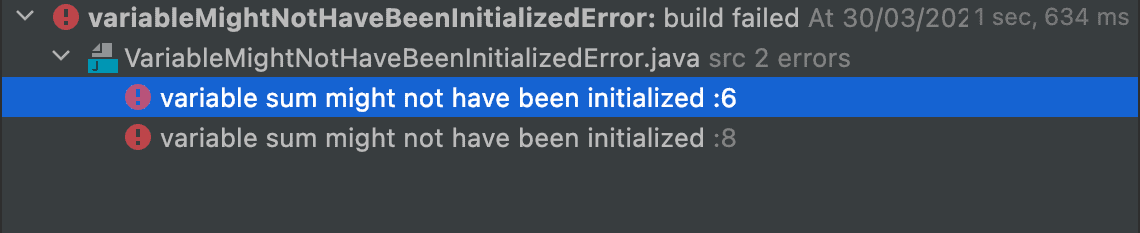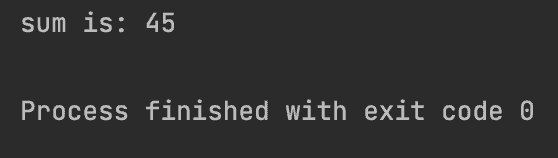## 1. Overview

In this tutorial, we’ll focus on the “variable might not have been initialized” error in Java programs. This error occurs when we declare a variable without initializing it. We’ll discuss this error with an example, and offer some solutions to solve it.

## 2. Java Error: “variable might not have been initialized”

If we declare a local variable without an initial value, we’ll get an error. This error occurs only for local variables, since Java automatically initializes the instance variables at compile time (it sets 0 for integers, false for boolean, etc.). However, local variables need a default value because the Java compiler doesn’t allow the use of uninitialized variables.

Let’s write a simple code having an uninitialized variable:

``````public class VariableMightNotHaveBeenInitializedError {
public static void main(String[] args) {
int sum;
int[] list = new int[]{1, 2, 3, 4, 5, 6, 7, 8, 9};
for (int i = 0; i < list.length; i++) {
sum += list[i];
}
System.out.println("sum is: " + sum);
}
}``````

In this code, we calculate the sum of a list of integer numbers. Then we put it in the variable sum. The following error appears at compile time:## 3. Solutions

To solve the error, we can simply assign a value to the variable when creating it:

``````public class VariableMightNotHaveBeenInitializedError {
public static void main(String[] args) {
int sum = 0;
int[] list = new int[]{1, 2, 3, 4, 5, 6, 7, 8, 9};
for (int i = 0; i < list.length; i++) {
sum += list[i];
}
System.out.println("sum is: " + sum);
}
}``````

Finally, by running the code, we get results without any errors:## 4. Conclusion

In this article, we discussed how uninitialized variables in Java cause errors. Then we wrote a simple Java code, and declared a local variable to hold the result of an operation without any errors.

### Get started with Spring and Spring Boot, through the Learn Spring course:

>> CHECK OUT THE COURSE